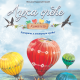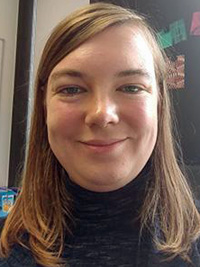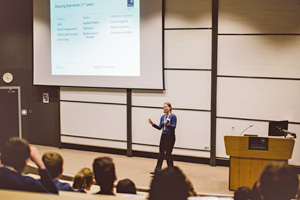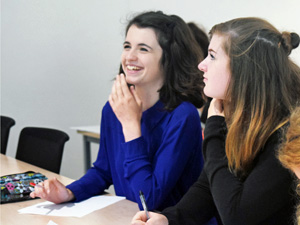Ово је портфолио Соње ШумоњаОДГОВОР РОДИТЕЉИМА КОЈЕ НЕ ЖЕЛЕ ОН ЛАЈН НАСТАВУДневник једне учитељице - Ивана Бошњак Бошњак

„Родитељи неће он лајн наставу“, бруји по свим медијима!

Чуј, родитељи неће!

Шта ће родитељима настава?

За њих није ни организована, ни осмишљена, него за децу.

Предлози тих истих родитеља су да деца „у мањим групама“ буду у учионици.

У којим мањим групама? Као, данас иду Пера, Мика и Жика, а сутра Мара, Сара и Лена?

Данас учим Перу, Мику и Жику, док остали ладе код куће, а сутра Мару, Сару и Лену, у среду Ђоку, Зокија и Угљешу? Шта раде остали ђаци код куће, док су „мање групе“ у школи?

Петицију потписало 1200 родитеља! Ехееј, 1200?! Дупло више их је само у мојој школи, а медији брује о „родитељима који неће…“

Кад 100 000 просветних радника нешто хоће или неће, тих истих медија нема као туриста на мору током пандемије!

Неки од тих родитеља држе малим Кинезима енглески он лајн. То је у реду. Мали Кинези могу да уче…

View original post 598 more words

S-E-E (Sun-Earth-Eratosthenes) New – Diseminacija projekta

Projekat gde sam jedan od autora uspešno živi svoj život!Математички коучинг

S-E-E (Sun-Earth-Eratosthenes) New

Opis projekta:

Students from all continents cooperate and collaborate to reproduce one of the science’s 10 most beautiful experiments of all time: Eratosthenes’ measurement of the Earth’s circumference. Although Eratosthenes experiment known for more than 22 centuries, it is always the preferable example for our students and for the implementation of the Inquiry method. Inquiry learning and active learner involvement can lead to important outcomes in the classroom. Students will also share knowledge about solar clocks and organize quizzes and competitions in the design and construction of solar clocks. We will inspire students and teachers to make mini-projects for the construction of solar clocks in the courtyards of the school, as well as the search for founders to fund the fabrication (entrepreneurship). The skills that students will develop are Lobbying and Advocacy.

„Učenici sa svih kontinenata saradjuju kako bi reprodukovali jedan od 10 najlepših eksperimenata nauke svih…

View original post 404 more words

This gallery contains 11 photos

Trigonometrijske jednačine i nejednačineZbirka zadataka

Trigonometrijske jednačine

1. Reši jednačinu: \$latex sin ^22x+sin ^2x=frac{3}{2}\$
2. Sva rešenja jednačine \$latex sin x+cos x+tan x=frac{1}{cos x}\$ su:
a) \$latex x=(2k+1)pi , kin Z\$;          b) \$latex x=kpi , kin Z\$;         c) \$latex x=2kpi , kin Z\$;         d) \$latex x=frac{pi }{2}+2kpi , kin Z\$;         e) \$latex x=frac{pi }{4}+2kpi ,kin Z\$.
3. Svih uglova \$latex x in left [ 0,2pi right ]\$ koji zadovoljavaju jednačinu \$latex 2 sin 3xtan 2x-tan 2x-2sin 3x+1=0\$:
a) \$latex 8\$;          b) \$latex 6\$;        c) \$latex 7\$;        d) \$latex 9\$;        e) \$latex 10\$.
4. Broj rešenja jednačine \$latex 1-cos (pi -x)+sin frac{pi +x}{2}=0\$ koja pripadaju intervalu \$latex left [ 1997 pi, 1998pi right ]\$ je:
a) \$latex 0\$;          b) \$latex 1\$;         c) \$latex 2\$;         d) \$latex 3\$;         e) veći od \$latex 3\$.
5. Zbir najvećeg i najmanjeg rešenja jednačine \$latex sqrt{3}-tan x=…

View original post 1.374 more words

Šta misle roditelji o nastavi na daljinu?KREATIVNA ČAROLIJA

U anonimnoj elektronskoj anketi učestvovalo je 2 632 roditelja iz 5 država, i to  52,2% roditelja učenika mlađih razreda, 39,9% roditelja učenika starijih razreda, 7% roditelja učenika srednjih škola, a ostalo su bili roditelji predškolaca.1.Na pitanje, da li njihovo dete pohađa nastavu na daljinu, njih 99,1% se izjasnilo pozitivno. To bi značilo, da manje od 1% učenika nije uključeno u nastavu na daljinu.2.Kroz pitanje, koji oblik nastave na daljinu koristi učitelj/nastavnik njihovog deteta, saznali smo da:

• 1% njih koristi samo komunikaciju putem FB
• 8,7 % koristi samo TV nastavu na daljinu,
• 12,7 % nastavnika koristi virtuelnu učinonicu na nekoj od brojnih platformi,
• 19 % njih koristi isključivo komunkaciju putem Vibera,
• 57, 6 % nastavnika/učitelja koristi miks više različitih oblika nastave na daljinu.Na osnovu ovih rezultata, jasno se vidi da oko 70 % nastavnika koristi nastavu na daljinu kombinujući virtelnu učionicu, nastavnu na daljinu, društevene mreže, dok…

View original post 1.158 more words

5G är inte ett mini-Tjernobyl i din ficka! Ta det lugnt och lär dig skillnaden mellan strålning och strålning!Konsten att flyga

Ibland önskar jag att folk läste in sig så pass mycket att de förstod de grundläggande skillnaderna mellan olika sorters elektromagnetisk strålning. Att all strålning inte automatiskt är skadlig.

Det finns joniserande strålning och icke-joniserande strålning. Joniserande strålning är av den sorten som har en så hög energi att den kan knocka loss elektroner från atomer, till exempel i ditt DNA, vilket betyder cancerrisk.

Exempel på sådan strålning är till exempel ultraviolett-, röntgen- och gammastrålning. De två sistnämnda har en sådan brutal energi att de dessutom går igenom stuff som en het kniv genom smör. Och slår som sagt loss elektroner.

Exempel på icke-joniserande strålning är radiofrekvent strålning, infraröd strålning och synligt ljus. Mobilstrålningen hör till den mer harmlösa radiofrekventa strålningen. Den är alltså som vanligt ljus, fast med en lite lägre frekvens, och en lägre energi.

Tumregeln lyder som så, att ju kortare våglängd -> desto…

View original post 918 more words

The Things That Mathematics Cannot ExplainThere are some mind blowing facts and games related to mathematics that even mathematics cannot explain. I just like to call those facts “The Abracadabra of Mathematics”.

Let’s take a look at one of those games or tricks. Assume that you are in a classroom with a population of at least 25 students and I am the instructor. I give a blank paper to each of you to write a digit , any number between 0–9, inclusive. After you are done writing your digit on your paper and fold it, I will collect the papers. Of course I don’t have any idea of what you write on the paper. However, I guarantee that I will know the most written number in the classroom.

Now, my claim is that most of the students chose the number 7. If a student comes and unfolds all of the papers and checks the papers, he would say, “You are correct! But how?” Unfortunately, I don’t have any explanation for this, though it is always true. Most people always choose number 7. I might have played this game more than 100 times, and I have never been wrong.

I learned this game from one of my favorite professors, Ali Nesin, 10 years ago. To play this game, there are some conditions. First, you need at least 25 people. Otherwise, it will be risky. You might think that this is about probability but actually it is not. Since there are 10 digits, the probability of each digit that is chosen is 1/10 for each student. So, the mathematical explanation is now working here. I think that this can also be explained with physiology or sociology.

There is also something which is extremely interesting that mathematics cannot explain. Here, we have 4 different rectangles. If we ask people which rectangle is more beautiful, 70%-80% would choose the green one.

We cannot also explain this situation using only mathematics because we don’t have a definition for beauty in mathematics, and this fact is mathematically incomprehensible. However, marketers used this information very effectively. When they realized that most people prefer a specific design.

After many years, we still couldn’t find an answer why people choose number 7, but an academician, Adrian Bejan found an answer as to why people choose the green rectangle. The professor discovered that “ the human eye is capable of interpreting an image featuring the golden ratio faster than any other.” So the green rectangle has the golden ratio and it seems more beautifully than the other rectangles.

You might’ve heard of Euclid. I have written some articles about him before. He had a book called “Elements”. I definitely recommend that you buy that book. In his book, “Elements”, Euclid defined the golden ratio as such:

A straight line is said to have been cut in extreme and mean ratio when, as the whole line is to the greater segment, so is the greater to the lesser.

In other words, Euclid was saying: There is a point on a segment such that we can call it the golden point which divides the line perfectly. His claim was assertive but also true.

Mathematically speaking, if you have a segment |AB|, and there exists a point C between the points A and B where we can get some ratios such as |AB|/|AC| and |AC|/|BC|. Then if this two ratios are equal, they are going to be golden ratio, 1.618…., φ (phi).

I am pretty sure that, this special ratio made you very very curious and you are wondering how Euclid got the value of the golden ratio? Let’s try to find it together.

Assume that:
the length of the |AC| = x and
the length of the |CB| = y. Then
That means if we can find the value of x/y we can find the value of φ and this will lead us to a quadratic equation.If we do cross multiply - (x+y) times y, and x times y - we get:                           x² = xy + y²Then we can combine all of the variables on one side and this time we get:                           x² - xy + y² = 0Reminder: our purpose is finding x/y. So if we divide all of the terms by y², then we get:
If we can define (x/y)= φ, we get:φ² — φ — 1 =0.Here, we need to remember the quadratic formula.The Quadratic Formula:Let a, b, and c be real number. The solutions of ax²+bx+c = 0 are
The quadratic formula tells us the product of the roots of our equation (c/a) is equal to -1. Thus, one the product of the roots is negative and the other one is positive. However, by the definition of the golden ratio, φ, cannot be negative. So we are going to choose the positive root. Now we can solve the equation.

So far we worked on a segment. We still need to show why people choose the green rectangle above and why Euclid called it the golden rectangle.

When we go back to our segment |AB| with the point C, we can fold it from the golden point C and get a right angle. So we can build a rectangle now. This rectangle is going to be the golden rectangle because the length of the sides will be x and y and we already showed that x/y is equal to the golden ratio, φ.

The golden rectangle has a property that no other rectangle has. This is a special rectangle such that, if you cut a square part from it, the leftover rectangle will be also a golden rectangle. I can leave this one as an exercise for you!

So far so good. Now, we can try something different such as find a golden triangle if there exists.

First of all, we need to decide what kind of triangle do we need to work on. Remember, when we remove a square part from a golden rectangle, we still have a golden rectangle. We need the same property for triangles. I think it is obvious that an equilateral triangle cannot be golden triangle because if you cut an equilateral triangle from an equilateral triangle the leftover part will not be an equilateral triangle.

However, I have good news, we can work on isosceles triangle! The steps will be clear. We will have an isosceles triangle and then we will cut another isosceles triangle from our original isosceles triangle and we will check if the leftover triangle is going to be similar to the original one or not. If yes, we will try to call it a golden triangle. I said we will try, because the next step will be finding the ratio of sides is equal to the golden ratio.

Let’s start with an isosceles triangle ABC with the base angles equal to 2α. Then we draw a line from the point B to the side |AC| to get two different isosceles triangles ABD and  BCD. Here we get something interesting because the base angles of the triangle BCD are also and the base angles of ABD are α because the sum of two interior angles will give us the exterior angle. So the angles of the triangle ABC is α, 2α, 2α. This gives us 5α = 180 and α = 36.
Now we get a very special triangle with top angle 36 and base angles 72.
For the second step, we need to check the ratio of the lengths of the triangle. If we say |AB| = |AC| = x and |BC| = y, then we get:|AD| = |BD| = y and |CD| = x-y.And our purpose is trying to find x/y = φ or not. By similarity, we get:

As you can see, we get the same quadratic equation at the end. So the triangle with the angles 36–72–72 deserves to be golden triangle. By the way, if you keep digging you will see that 108–36–36 is also a golden triangle. This information will be very useful when we deal with a pentagon.

Let’s do an example. If you have a pentagon, what is the ratio between the length of its diagonal and the length of its side?If you draw a diagonal from any edge we will get a triangle which is a golden triangle because the angles will be 108, 36, and 36.So, if the length of one side of the pentagon is 1, then the length of the diagonal will be φ.

We solve a challenge question without doing any math. Without any knowledge of the golden ratio, we have to deal with a lot of lines, quadratic equations, etc…

Let’s do another example. If we draw all the diagonals of a pentagon we will get something unique. There will be smaller pentagon in the middle and every triangle you see is going to be a golden triangle.
Let’s do another example. If we draw all the diagonals of a pentagon we will get something unique. There will be smaller pentagon in the middle and all the triangles you see is going to be a golden triangle.Here is the question: what is the ratio between the area of the small pentagon and the area of the big one?We can easily do it because if we call one side of the small pentagon 1, the length of the other side of the small triangles will be φ. Then the same ratio will tell us the base length of the triangle will be φ². Now the similarity will give us the ratio. The similarity ratio is 1/φ², then the ratio of the areas will be 1/φ⁴.

There is also another unique property that φ has. If we go back, we will remember the quadratic equation of φ.

φ² — φ — 1 = 0, so this will give us:φ² = φ +1

φ is the only number which its square is equal to the sum of itself and 1. So there is no real number such that when you add 1 that you get square of that real number. And interestingly enough, we can have something like this:

φ² = 1φ + 1φ³ = 2φ + 1φ⁴ = 3φ + 2φ⁵ = 5φ + 3φ⁶ = 8φ + 5...

Here the constant numbers are interesting. 1, 1, 2, 3, 5, 8, 13, 21, 34, 55, …. These are not random numbers. These numbers are coming from the Fibonacci sequence where each term is the sum of the previous two terms.

The relation between Fibonacci sequence and the golden ratio is unique. The ratio of 2 consecutive numbers from the sequence is getting the golden ratio after a while. You are getting every digit of golden ratio if you deal with big number from the sequence.

For instance 5/3= 1.666…8/5= 1.613/8 = 1.61…21/13 = 1.618…

If you keep doing this you will get a new digit of a φ.

This information is very useful because we can also find sin 18 or cos 36 easily without a calculator. This is also exercise for you!

https://medium.com/however-mathematics/the-things-that-mathematics-cannot-explain-a9e5ab3ae96a

Dr. Rebecca Cotton-Barratt, Lecturer in Mathematics at Christ Church College, University of OxfordDr. Rebecca Cotton-Barratt is a lecturer in mathematics at Christ Church, one ofthe largest colleges of the University of Oxford. She was an earth sciences undergraduate at Hertford College, Oxford before moving to the University of Warwick to complete a Ph.D. in mathematics. Rebecca is also the undergraduate admissions coordinator for the Mathematical Institute and she helps to run their extensive outreach programme.“…there are a lot of similarities between maths and sport – people get put off both of them by having horrible experiences at school, or thinking that they just can’t do it, and then giving up…”

From dinosaurs to mathematics

At school mathematics definitely made sense to me as a subject (I think I even had a brief period where I wanted to be a maths professor), but then I became interested in dinosaurs and prioritised that more instead of maths. I took maths A-level (but not Further Maths – I was already doing five A-levels and there wasn’t enough room to fit in a sixth!), and then I studied earth sciences at the University of Oxford.

It was definitely during my degree that I became more switched on to maths – I enjoyed the geophysics options and linear algebra, as well as the statistical analysis around palaeontology, and then one of my tutors introduced me multi-dimensional morphospaces and I was hooked! Being friends with lots of mathematicians during my undergraduate probably helped nudge me in that direction too.

After my undergraduate degree I wanted to do something more theoretical, so I did a Ph.D. in mathematics and complexity science at the University of Warwick, looking at mathematical models of evolution (combining my two loves – maths and evolution!).

No need to be pigeon-holedI don’t have a typical day – my work is very seasonal, so one day I might be talking to 300 sixth formers, the next processing 4000 admissions test scripts. I run and help to organise lots of events for school students throughout the year, both maths-specific and multi-subject focused.

One of the big messages I try to get across is that although it’s important to study the right subject for you at degree level, you shouldn’t let that constrain you later on in your career – there’s so much movement between different disciplines within STEM, but we grow up with this quite artificial grouping of “this is maths”and “this is biology / physics / science”.

When I’m not doing outreach, I’m coordinating the undergraduate admissions process for mathematics at Oxford, which involves lots of operational thinking to help tutors make the best admissions decisions.

Fighting against the societal perception of mathematics

The 4000 admissions test scripts are amongst my greatest challenges. We mark them all in four days, so there’s a very fast turn around and the process has to be watertight. That’s an operational challenge, but I think the biggest outreach and admissions challenge is fighting against the societal perception of mathematics.In the UK less than 30% of the students who take Further Maths A-level are women and Further Maths A-level didn’t even appear in the list of A-level subjects African and Caribbean students are taking. When I started digging into admissions data I was asking the question “what proportion of the potential pool of students are we already attracting?” and what I found was that we were already attracting over 20% of the national pool of women who achieve an A* in Further Maths at A-level.

So, if you’re trying to tackle under-representation of groups at degree level, it’s not enough to work with sixth formers – you have to start increasing the pool of potential applicants by working with younger students and informing them about A-level choices.

Similarities between experiences of maths and sport at school

There’s been a lot of really good research done about growth mind set and mathematics, and I think that message is starting to get through to both teachers and students. I think there are a lot of similarities between maths and sport – people get put off both of them by having horrible experiences at school, or thinking that they just can’t do it, and then giving up.It used to be the case that only high-profile sports people were visible – so if you couldn’t be a gold medallist, what was the point? I think maths is still viewed like that – people think you have to be you have to be on track to win a Fields Medal in order to continue studying it (and one of the proxies we have for measuring that is how easy you find maths – which feeds this genius myth).

With sport however, there’s starting to be this great community (like Couch to 5kand the This Girl Can campaign) which have started to make exercise more accessible. I’d really like to see something similar happen for mathematics in my lifetime. I have a dream of people doing maths for pleasure, much like people read or exercise for pleasure.

Career options for mathematicians

I genuinely believe that mathematics is the best subject to study if you’re not sure what you want to do. Mathematicians go into such a range of careers after graduating – some directly use their maths (like in finance or accountancy) but others go into careers that don’t require a maths background at all (but might draw on the problem-solving skills that a maths degree teaches you).

There’s so much data-driven analysis now going on in different sectors that quantitative skills are useful in any sector.

My advice for career changers and mature students is that one of the things you bring to the table is outside perspective and experience so use those to your advantage. Think about where maths could have been used in your previous area and make connections between different ideas. Don’t worry when things aren’t obvious – think about them until they make sense internally to you.